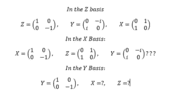# Pauli Matrices in the Basis of Y?

Destroxia

## Homework Statement

[/B]
I know the pauli matrices in terms of the z-basis, but can't find them in terms of the other bases. I would like to know what they are.

## Homework Equations

The book says they are cyclic, via the relations XY=iZ, but this doesn't seem to apply when I use this to find the basis states.

## The Attempt at a Solution

I understand the idea of changing basis, I just need to see what the pauli matrices are in the Y basis so I can finally confirm it. I have looked through books, and webpages, and they only give them in the standard basis for Z.I have been looking at this forever... if in the X basis the Z matrix is just the same as the X matrix in the Z basis, doesn't that just leave the Y matrix in the X basis the same as in the Z basis?

And if that's true, where does that leave the X matrix and Z matrix in the Y basis?

Homework Helper
Gold Member
2022 Award
You can do this either by appealing to symmetry, or as an exercise you could find a change of basis matrix - from Z to Y - and apply that to the three matrices.

First, from symmetry, what is ##\sigma_y## in the Y basis?

Destroxia
You can do this either by appealing to symmetry, or as an exercise you could find a change of basis matrix - from Z to Y - and apply that to the three matrices.

First, from symmetry, what is ##\sigma_y## in the Y basis?

I'm not sure what the matrix is for Z to Y, I can't figure it out and the textbook doesn't list anything besides X to Z.

and I believe that ##\sigma_y## in the Y basis is the same as ##\sigma_z## in the Z basis.

## \sigma_y = \left(\begin{array}{cc}1&0\\0&-1\end{array}\right)## in the Y basis.

Homework Helper
Gold Member
2022 Award
and I believe that ##\sigma_y## in the Y basis is the same as ##\sigma_z## in the Z basis.

## \sigma_y = \left(\begin{array}{cc}1&0\\0&-1\end{array}\right)## in the Y basis.

Yes, exactly. Now, you need to work out the correct order for the other two. The key is the base permutation ##x,y,z##.

Homework Helper
Gold Member
2022 Award
PS you also might want to figure some notation for this. First, perhaps, use ##\sigma_1, \sigma_2, \sigma_3## for the underlying Pauli matrices. Then something like ##\sigma_{Z,x}## for the x-matrix in the Z basis.

Destroxia
Yes, exactly. Now, you need to work out the correct order for the other two. The key is the base permutation ##x,y,z##.

I read in the book a cyclic relation, is this what you mean by base permutation? ## XY=iZ ##, ## ZX=iY ##, ## YZ=iX ##... Does this mean that I can simply equate them so that ## \sigma_y = \left(\begin{array}{cc}0&-i\\i&0\end{array}\right) ## in the X basis, and ## \sigma_y = \left(\begin{array}{cc}0&1\\1&0\end{array}\right) ## in the Z basis?

Destroxia
PS you also might want to figure some notation for this. First, perhaps, use ##\sigma_1, \sigma_2, \sigma_3## for the underlying Pauli matrices. Then something like ##\sigma_{Z,x}## for the x-matrix in the Z basis.

Also, I apologize I got your note on notation after I submitted that.

Homework Helper
Gold Member
2022 Award
I read in the book a cyclic relation, is this what you mean by base permutation? ## XY=iZ ##, ## ZX=iY ##, ## YZ=iX ##... Does this mean that I can simply equate them so that ## \sigma_y = \left(\begin{array}{cc}0&-i\\i&0\end{array}\right) ## in the X basis, and ## \sigma_y = \left(\begin{array}{cc}0&1\\1&0\end{array}\right) ## in the Z basis?

You should already know that the three matrices in the Z-basis, so that last statement is wrong. In fact, they are both wrong.

The idea is simply that x,y,z have a certain order. You know that in the Z basis the order is ##z,x,y## and this maps to ##1,2,3##.

So, in the X basis the order is ##x,y,z## and that, by symmetry, must equate to ##1,2,3##.

In general, going from Z to X, replace z by x, x by y and y by z.

Does that make sense?

Homework Helper
Gold Member
2022 Award
PS that's what is meant by a cyclic relation. The order is always x, y, z whichever one you start with. It's never x, z, y, which is the opposite cycle.

PPS in physical terms this relates to the orientation of the axes. The opposite cycle would apply if we had the z-axis pointing down.

Last edited:
Destroxia
PS that's what is meant by a cyclic relation. The order is always x, y, z whichever one you start with. It's never x, z, y, which is the opposite cycle.

So if I understand you correctly...

## \sigma_{y,y} = \left(\begin{array}{cc}1&0\\0&-1\end{array}\right) ##, ## \sigma_{y,z} = \left(\begin{array}{cc}0&1\\1&0\end{array}\right) ##, ## \sigma_{y,x} = \left(\begin{array}{cc}0&-i\\i&0\end{array}\right) ##

In which the first "sub" term is the y basis, and the 2nd "sub" term is the corresponding matrix?